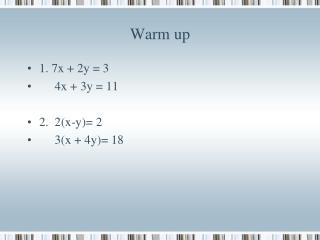DownloadDownload PresentationWarm up

# Warm up

Download Presentation## Warm up

- - - - - - - - - - - - - - - - - - - - - - - - - - - E N D - - - - - - - - - - - - - - - - - - - - - - - - - - -
##### Presentation Transcript

1. Warm up • 1. 7x + 2y = 3 • 4x + 3y = 11 • 2. 2(x-y)= 2 • 3(x + 4y)= 18

2. Lesson 9-6 Wind & Water Current Problems Objective: To use systems of equation to solve wind & water current problems.

3. Current Problems • Distance = Rate x Time • r = rate of the boat/plane • w = wind/water current • with the current r + w • against the current r - w

4. Example • A plane can fly 3750 km in 3 h with the wind. The plane takes 5 h to travel the same distance flying against the wind. Find the rate of the plane in still air and the speed of the wind. • 3(r + w) = 3750 r + w = 1250 • 5(r – w) = 3750 r – w = 750 • 2r = 2000 r = 1000, w = 250

5. Example • Brad can row a boat 30 km downstream in 3 h, but it takes him 5 h to return. What is Brad's rate in still water? What is the rate of the current?